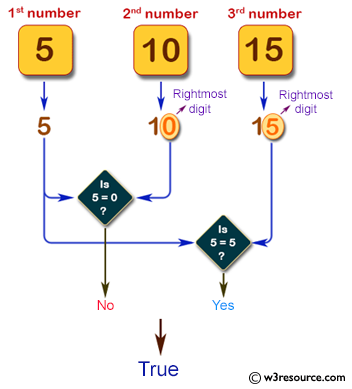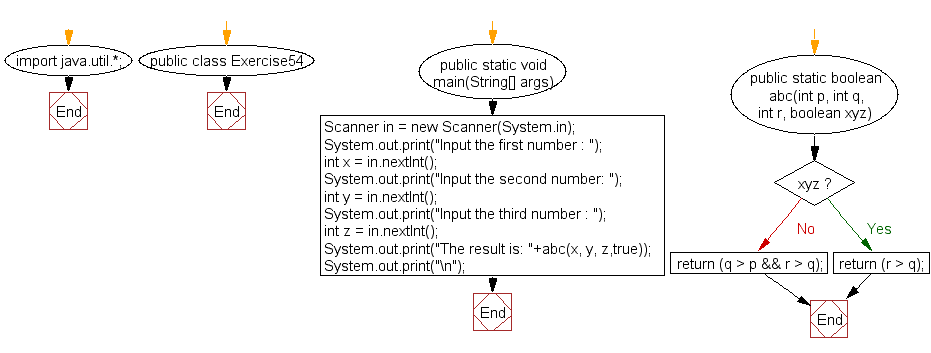﻿ Java exercises: Accepts three integers from the user and return true if two or more of them have the same rightmost digit - w3resource# Java Exercises: Accepts three integers from the user and return true if two or more of them have the same rightmost digit

## Java Basic: Exercise-54 with Solution

Write a Java program that accepts three integers from the user and return true if two or more of them (integers ) have the same rightmost digit. The integers are non-negative.

Sample Solution:

Java Code:

``````import java.util.*;
public class Exercise54 {
public static void main(String[] args)
{
Scanner in = new Scanner(System.in);
System.out.print("Input the first number : ");
int x = in.nextInt();
System.out.print("Input the second number: ");
int y = in.nextInt();
System.out.print("Input the third number : ");
int z = in.nextInt();
System.out.print("The result is: "+test_last_digit(x, y, z,true));
System.out.print("\n");
}

public static boolean test_last_digit(int p, int q, int r, boolean xyz)
{
return (p % 10 == q % 10) || (p % 10 == r % 10) || (q % 10 == r % 10);
}
}
```
```

Sample Output:

```Input the first number : 5
Input the second number: 10
Input the third number : 15
The result is: true
```

Pictorial Presentation:Flowchart:Java Code Editor:

What is the difficulty level of this exercise?

﻿

## Java: Tips of the Day

Execution of console commands:

Java allows you to execute console commands directly from code using a class Runtime. It is very important not to forget about exception handling.

For example, let's try to open a PDF file through a Java terminal:

```import java.io.BufferedReader;
import java.io.InputStream;

public class ShellCommandExec {

public static void main(String[] args) {
String gnomeOpenCommand = "gnome-open //home//user//Documents//MyDoc.pdf";

try {
Runtime rt = Runtime.getRuntime();
Process processObj = rt.exec(gnomeOpenCommand);

InputStream stdin = processObj.getErrorStream();

String myoutput = "";